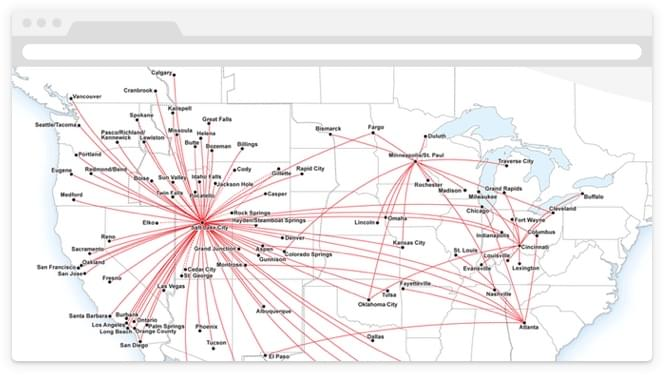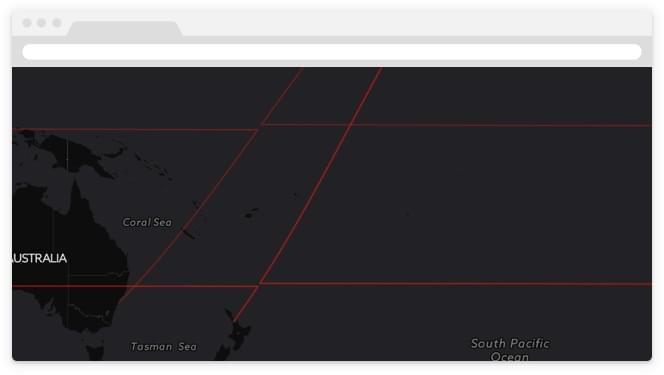# Flying Around the World with CartoDB

Summary

This post may describe functionality for an old version of CARTO. Find out about the latest and cloud-native version here.As a child nothing was more exciting to me than a chance to ride on an airplane. And after enjoying playing with the seatbelt buckle and feeling the crazy push of take-off acceleration I would usually settle in and page to the back of the in-flight magazine where the airline route maps were: where were we going today and where could we go tomorrow?

### Destination Map

We can build route maps for any city in the world using airport and route data from OpenFlights.org. Start by uploading the airports.csv and routes.csv files into CartoDB.

We can see every destination available starting from Vancouver Canada (airport code "YVR") by making some custom SQL to join the `airports` table to the `routes` table and restricting to just the "YVR" routes:

##_INIT_REPLACE_ME_PRE_##

```SELECT a2.cartodb_id  a2.the_geom_webmercator  a2.city  r.airline
FROM airports a1
JOIN routes r ON r.airport_st = a1.code_iata
JOIN airports a2 ON r.airport_end = a2.code_iata
WHERE a1.code_iata = 'YVR' AND r.codeshare IS NULL
{% endhighlight sql %}```

That's the data we want but without the flight lines it lacks a sense of movement.

### Simple Route Map

Our query is already joining the airports twice: once for the origin and once for the destination airport so we can turn the end points into a line very easily using the ST_MakeLine() function:

##_INIT_REPLACE_ME_PRE_##

```SELECT a2.cartodb_id
a2.city  r.airline
ST_Makeline(a2.the_geom_webmercator  a1.the_geom_webmercator) as the_geom_webmercator
FROM airports a1
JOIN routes r ON r.airport_st = a1.code_iata
JOIN airports a2 ON r.airport_end = a2.code_iata
WHERE a1.code_iata = 'YVR' AND r.codeshare IS NULL
{% endhighlight sql %}```

First the routes are all straight lines and they should be great circle routes that's how the airplanes fly!

Second some of the routes go the wrong way around the world: no airline would fly from Vancouver to Sydney via Africa!

### Great Circle Route Map

If we convert our lines into great circle routes we can maybe kill both of these birds with one stone since the great circle routes will go the right direction.

##_INIT_REPLACE_ME_PRE_##

```SELECT a2.cartodb_id
a2.city AS city  r.airline
ST_Transform(
ST_Segmentize(
ST_Makeline(
a2.the_geom
a1.the_geom
)::geography
100000
)::geometry
3857
) as the_geom_webmercator
FROM airports a1
JOIN routes r ON r.airport_st = a1.code_iata
JOIN airports a2 ON r.airport_end = a2.code_iata
WHERE a1.code_iata = 'YVR' AND r.codeshare IS NULL
{% endhighlight sql %}```

This is a bit complex but reading the nested functions outwards starting from the `ST_MakeLine()` we:

• Cast the point-to-point line to the "geography" type which understands edges (also known as connections between nodes) as great circles; then
• Use the `ST_Segmentize(geography)` function to add points to the line along the great circle (so when we put it back on the flat map it'll appear curved); then
• Cast the line back into the "geometry" type we use for flat mapping; and finally
• Transform the coordinates of the line using `ST_Transform(geometry srid)` to the web mercator projection we use on our flat maps.

The end result is really really close!

But what is going on with those horizontal lines?

### Great Circle Route Map with Dateline Fix

There's a gap right where the horizontal line appears.Everything is fine until an edge on the great circle route tries to cross the dateline. Then it zings around the world in order to hook up to the next edge. Fundamentally our map still does not understand the circularity of the world even though the edges we built do understand it. We have to work around the limitations of the flat map by chopping our data at the dateline to avoid having edges that cross it.

##_INIT_REPLACE_ME_PRE_##

```-- First build our lines just as before  this can be any raw data you
-- need to feed into a dateline chopping process
WITH lines AS (
SELECT a2.cartodb_id
a2.city  r.airline
ST_Segmentize(ST_Makeline(a2.the_geom  a1.the_geom)::geography 100000)::geometry::geography::geometry as the_geom
FROM airports a1
JOIN routes r ON r.airport_st = a1.code_iata
JOIN airports a2 ON r.airport_end = a2.code_iata
WHERE a1.code_iata = 'YVR' AND r.codeshare IS NULL
)
-- Now break the input data into two sets  one to split and one to leave
-- unprocessed. Objects that cross the dateline will appear to be very wide
-- (as they zing across the world) so we'll only chop features that are very
-- wide. This is just for efficiency.
tosplit AS (
SELECT * FROM lines
WHERE ST_XMax(the_geom) - ST_XMin(the_geom) > 180
)
-- Narrow objects we'll leave un-chopped.
nosplit AS (
SELECT * FROM lines
WHERE ST_XMax(the_geom) - ST_XMin(the_geom) <= 0="" 90="" 180="" 360="" )="" --="" in="" order="" to="" chop="" the="" objects="" we="" need="" get="" them="" into="" a="" space="" where="" they="" make="" "sense"="" so="" shift="" any="" vertex="" that="" is="" <="" right="" by="" units="" effectively="" building="" map="" ranging="" from="" centered="" around="" dateline="" instead="" of="" usual="" -180="" at="" greenwich.="" then="" if="" split="" nice="" cut="" just="" want="" it.="" removing="" very="" narrow="" gap="" wide="" enough="" don't="" wee="" rounding="" errors="" dateline.="" as="" (="" select="" cartodb_id="" city="" airline="" st_difference(st_shift_longitude(the_geom)="" st_buffer(st_geomfromtext('linestring(180="" -90)'="" 4326)="" 0.00001))="" the_geom="" tosplit="" merge="" features="" back="" with="" unprocessed="" features.="" final="" *="" union="" all="" nosplit="" transform="" web="" mercator="" for="" mapping.="" projection="" handles="" longitudes=""> 180 as if they were
-- negative longitudes  so there is no need to convert them back.
SELECT
cartodb_id
city  airline
ST_Transform(the_geom 3857) AS the_geom_webmercator
FROM final
{% endhighlight sql %}```

And it works!

Of course this is a route map of all flights leaving Vancouver (YVR) so it's not exactly the kind of map you'd find in a in-flight magazine. However it's easy to build such a map just by changing set of input airports we use to build the routes.

Where the existing query says:

##_INIT_REPLACE_ME_PRE_##

``` WHERE a1.code_iata = 'YVR' AND r.codeshare IS NULL
{% endhighlight sql %}```

Replace the airport filter with an airline filter of "AC" to get an Air Canada route map:

##_INIT_REPLACE_ME_PRE_##

``` WHERE r.airline = 'AC' AND r.codeshare IS NULL
{% endhighlight sql %}```

Or try "UA" for a United map or "DL" for a Delta map.

Happy flying!